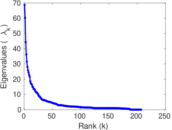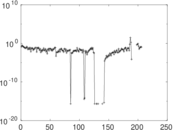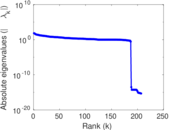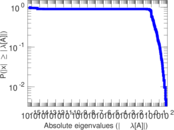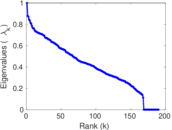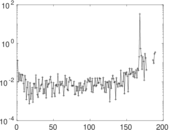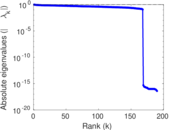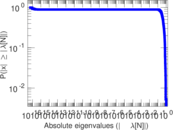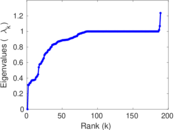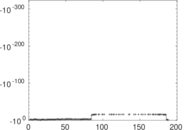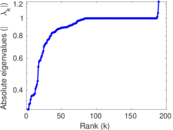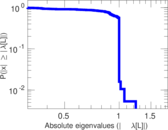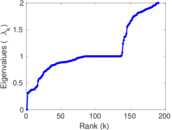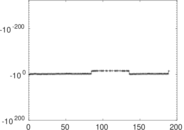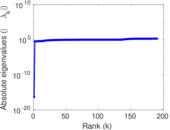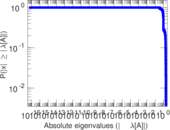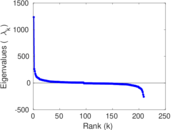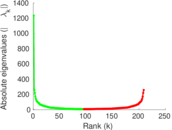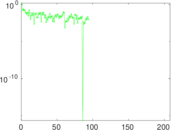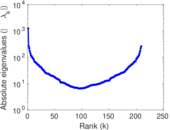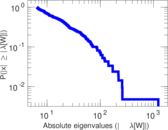This is the bipartite edit network of the Adyghe Wikipedia. It contains users and pages from the Adyghe Wikipedia, connected by edit events. Each edge represents an edit. The dataset includes the timestamp of each edit.

 Code `ady` Internal name `edit-adywiki` Name Wikipedia edits (ady) Data source http://dumps.wikimedia.org/ AvailabilityDataset is available for download Consistency checkDataset passed all tests Category Authorship network Dataset timestamp 2017-10-20 Node meaning User, article Edge meaning Edit Network formatBipartite, undirected Edge typeUnweighted, multiple edges Temporal dataEdges are annotated with timestamps

## Statistics

 Size n = 1,254 Left size n1 = 209 Right size n2 = 1,045 Volume m = 6,003 Unique edge count m̿ = 3,969 Wedge count s = 515,670 Claw count z = 79,797,409 Cross count x = 11,228,797,952 Square count q = 337,370 4-Tour count T4 = 4,776,850 Maximum degree dmax = 676 Maximum left degree d1max = 676 Maximum right degree d2max = 111 Average degree d = 9.574 16 Average left degree d1 = 28.722 5 Average right degree d2 = 5.744 50 Fill p = 0.018 172 7 Average edge multiplicity m̃ = 1.512 47 Size of LCC N = 1,190 Diameter δ = 8 50-Percentile effective diameter δ0.5 = 2.434 22 90-Percentile effective diameter δ0.9 = 3.892 18 Median distance δM = 3 Mean distance δm = 3.046 81 Gini coefficient G = 0.694 123 Balanced inequality ratio P = 0.239 963 Left balanced inequality ratio P1 = 0.124 771 Right balanced inequality ratio P2 = 0.321 839 Relative edge distribution entropy Her = 0.812 040 Power law exponent γ = 1.913 96 Tail power law exponent γt = 2.701 00 Tail power law exponent with p γ3 = 2.701 00 p-value p = 0.000 00 Left tail power law exponent with p γ3,1 = 1.621 00 Left p-value p1 = 0.221 000 Right tail power law exponent with p γ3,2 = 3.901 00 Right p-value p2 = 0.067 000 0 Degree assortativity ρ = −0.174 358 Degree assortativity p-value pρ = 1.834 63 × 10−28 Spectral norm α = 68.678 7 Algebraic connectivity a = 0.312 324 Spectral separation |λ1[A] / λ2[A]| = 1.140 08 Controllability C = 857 Relative controllability Cr = 0.696 182

## Plots

### Fruchterman–Reingold graph drawing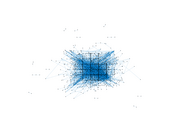### Degree distribution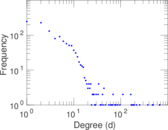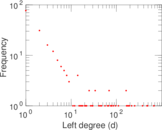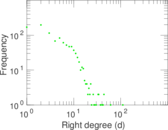### Cumulative degree distribution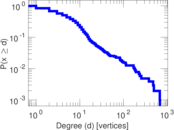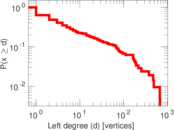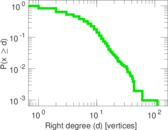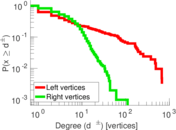### Lorenz curve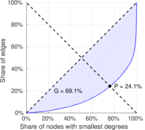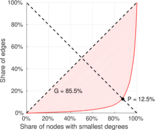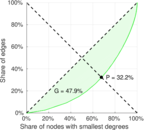### Spectral distribution of the adjacency matrix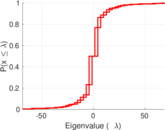### Spectral distribution of the normalized adjacency matrix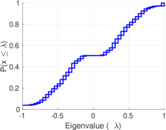### Spectral distribution of the Laplacian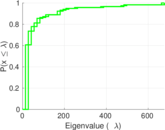### Spectral graph drawing based on the adjacency matrix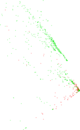### Spectral graph drawing based on the Laplacian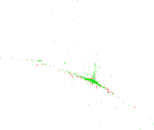### Spectral graph drawing based on the normalized adjacency matrix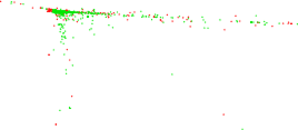### Degree assortativity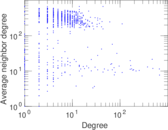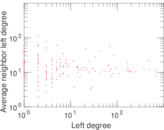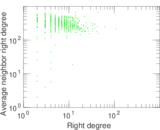### Zipf plot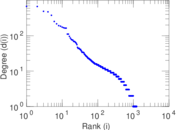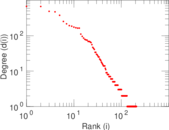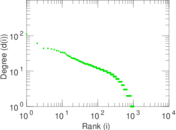### Hop distribution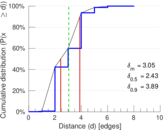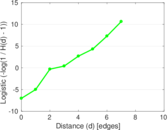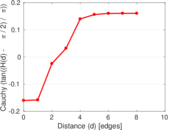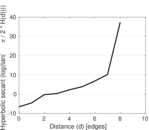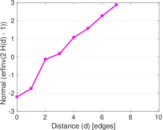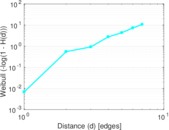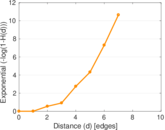### Double Laplacian graph drawing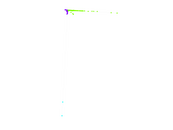### Delaunay graph drawing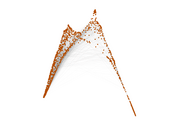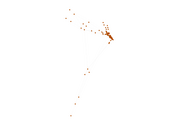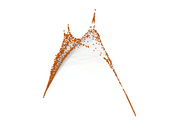### Edge weight/multiplicity distribution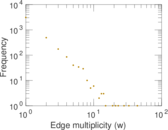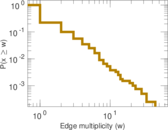### Temporal distribution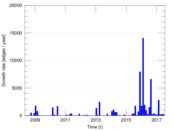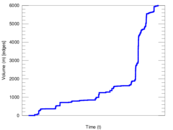### Temporal hop distribution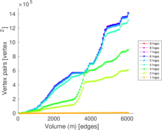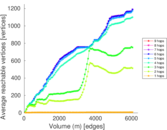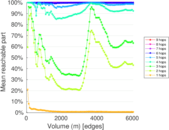### Diameter/density evolution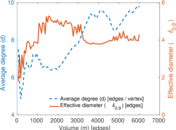### Matrix decompositions plots